Next: 5.4 Boundaries Up: 5.3 Interfaces Previous: 5.3.1 Neumann Type (Explicit

## 5.3.2 Dirichlet Type (Implicit Flux)

In case an expression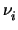= h(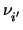) is available F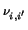can be eliminated by adding (5.9) to (5.10) and the resulting equations are of the following form:
 f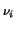=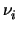- h(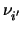) = 0 (5.13) f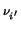= fS+ fS= 0 (5.14)

As (5.13) is normally not diagonal-dominant it is eliminated in a pre-pass. It is important to note, that in this case the structure of the equation system changes. The constitutive relation foris now given by (5.13) whereas forby (5.14), or vice-versa. This is accomplished by the following transformation matrix

 tx, y1 1

The fluxes contained in fcan also be used to calculate the total interface flux FI by setting up the following equation:

 f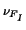=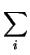fS+ FI = 0 (5.15)

with i running over all interface points.Next: 5.4 Boundaries Up: 5.3 Interfaces Previous: 5.3.1 Neumann Type (Explicit
Tibor Grasser
1999-05-31